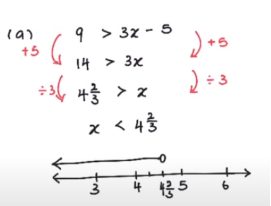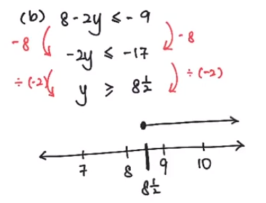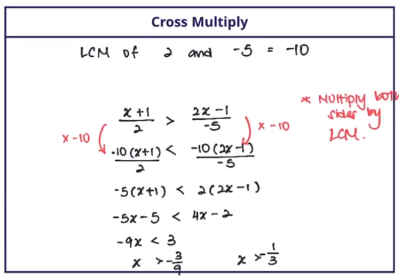Study S3 Mathematics Linear Inequalities - Geniebook# Linear Inequalities

• Solving Linear Inequalities
• Illustrating solutions on a number line.

• Solving Simultaneous Linear Inequalities
• Illustrating solutions on a number line.

## Solving Linear Inequalities

Example 1:

Solve $$x + 4 > 10$$ and illustrate the solution on a number line.

Solution:

 \begin{align*} x + 4 &> 10 \\[2ex] x &> 10-4 \\[2ex] x &> 6 \end{align*}When solving for linear inequalities, remember that if we multiply both sides of the inequality by a negative number, the inequality sign is reversed.

Example 2:

Solve \begin{align*} -\frac {x}{2} \geq 4 \end{align*} and illustrate the solution on a number line.

Solution:

 \begin{align*} -\frac {x}{2} &\geq 4 \\[2ex] &\text {Upon multiplying both sides by -2, we get,} \\[2ex] x &≤ -8 \end{align*}Example 3:

Solve \begin{align*} -3x ≤ 15 \end{align*} and illustrate the solution on a number line.

Solution:

 \begin{align*} -3x &≤ 15 \\[2ex] &\text {Dividing both sides by (-3) we get,} \\[2ex] x &\geq - 5 \end{align*}Question 1:

Solve each of the following inequalities and illustrate them on the number line.

a)  $$9 > 3x-5$$

Solution:

\begin{align*} 9 &> 3x - 5 \\ 14 &> 3x \\ \frac{14}{3} &> x & \text {(Dividing by 3 on both sides)} \\ x &< 4\frac{2}{3} \end{align*}b) \begin{align*} 8 - 2y ≤ -9 \end{align*}

Solution:

\begin{align*} 8 - 2y &≤ -9 \\ -2y &≤ -9 -8 \\ -2y &≤ -17 \\ y &\geq \frac{17}{2} & \text {(Divided by 2 and multiplied by - sign on both sides )} \\ y &\geq 8\frac{1}{2} \end{align*}## Investigation Of Concept

How do we solve \begin{align*} \frac {x+1}{2} =\frac {2x-1}{-5} \end{align*} ?

Upon cross multiplying the two equations we get,

\begin{align*} -5(x+1) &= 2(2x-1) \\ -5x-5 &= 4x-2 \\ -9x &= 3 \\ x &= -\frac {1}{3} \end{align*}

But then,

How do we solve \begin{align*} \frac {x+1}{2} >\frac {2x-1}{-5} \end{align*} ?

Take the LCM of $$2$$ and $$-5$$, we get $$-10$$.

Multiply both sides with $$-10$$,

\begin{align*} \frac {-10(x+1)}{2} &< \frac {-10(2x-1)}{-5} \\ -5(x+1) &< 2(2x-1) \\ -5x-5 &< 4x-2 \\ -9x &<3 \\ x &> -\frac {1}{3} \end{align*}Question 2:

Solve each of the following inequalities

1) \begin{align*} \frac {x+3}{2} >\frac {2x-1}{5} \end{align*}

Solution:

Take the LCM of $$2$$ and $$5 = 10$$,

Multiply both sides with $$10$$,

\begin{align*} \frac {10(x+3)}{2} &< \frac {10(2x-1)}{5} \\ 5(x+3) &< 2(2x-1) \\ 5x+15 &< 4x-2 \\ 5x-4x &<15-2 \\ x &< -17 \end{align*}

2) \begin{align*} -\frac {2x+1}{4} \geq\frac {6-x}{3} \end{align*}

Solution:

Take the LCM of $$3$$ and $$​4 =12 ​$$,

Multiply both sides with $$12$$,

Note: \begin{align*} -\frac {2x+1}{4} \end{align*} can be written as \begin{align*} \frac {2x+1}{-4} \end{align*} or \begin{align*} \frac {-2x-1}{4} \end{align*}

\begin{align*} -\frac {12(2x+1)}{4} &\geq\frac {12(6-x)}{3} \\ -3(2x+1) &\geq 4(6-x) \\ -6x-3 &\geq 24-4x \\ -6x+ 4x &\geq 24+3 \\ -2x &\geq 27 \\ x &\leq-13\frac{1}{2} \end{align*}

## Simultaneous Linear Inequalities

Let us take a few examples to understand the notion of simultaneous linear inequalities.

Simultaneous Linear inequalities essentially contain two equations which when solved at the same time, the solution of the two equations lies on those points where their individual solutions are common, when charted on a number line.

Example 4:

Find the range of values of $$x$$ for which $$x<1$$ & $$x>5$$?

Solution:

Upon charting them on the number line we obtain,Since the two solutions do not overlap with each other at any point, there is no solution.

Example 5:

Find the range of values of $$x$$ for which $$x>1$$ & $$x>5$$?

Solution:

Upon charting them on the number line we obtain,The obtained graph shows that there is a region of overlap at $$x>5$$ and hence, that is the solution of the simultaneous linear inequality.

Question 3:

Find the range of values of $$x$$ for which $$-2x<5-x$$ & $$-4x-2\geq-3x+1$$

Illustrate the solution on the number line.

Solution:

For Equation 1:

\begin{align*} -2x &< 5-x \\ -2x+5 &< 5 \\ -x &< 5 \end{align*}

Multiplying $$-1$$ on both sides,

$$\implies x > -5$$

For Equation 2:

\begin{align*} -4x-2 &\geq -3x+1 \\ -4x+3x &\geq 1+2 \\ -x &\geq 3 \end{align*}

Multiplying by $$-1$$ on both sides,

$$\implies x \leq -3$$

Hence the final solution is $$-5 < x \leq -3$$Question 4:

Solve the inequalities $$3x+8<-x-3<\frac {1}{2}x-9.$$

Solution:

The above equation can be split and written as $$3x+8<-x-3$$ & $$-x-3<\frac {1}{2}x-9$$ separately.

For Equation 1:

\begin{align*} 3x+8 &< -x-3 \\ 3x+x &< -8-3 \\ 4x &< -11 \\ x &<-2\frac {3}{4} \end{align*}

For Equation 2:

\begin{align*} -x-3 &< \frac{1}{2}x-9 \\ -x-\frac{1}{2}x &< -9+3 \\ -1\frac{1}{2}x &< -6 \end{align*}

Multiplying both sides with $$-\frac{2}{3}$$

$$\implies x> 4$$Since there is no overlap between the two solutions, there is no solution.

## Conclusion

In this article, we learnt about solving linear inequalities problems and the concept of solving Simultaneous Linear Equations.

Continue Learning
Further Trigonometry Quadratic Equations And Functions
Linear Inequalities Laws of Indices
Coordinate Geometry Graphs Of Functions And Graphical Solution
Applications Of TrigonometryPrimaryPrimary 1Primary 2Primary 3Primary 4Primary 5Primary 6SecondarySecondary 1Secondary 2Secondary 3PhysicsChemistryMathsE-Maths
Further Trigonometry
Linear Inequalities
Laws Of Indices
Coordinate Geometry
Graphs Of Functions And Graphical Solution
Applications Of TrigonometryA-MathsSecondary 4